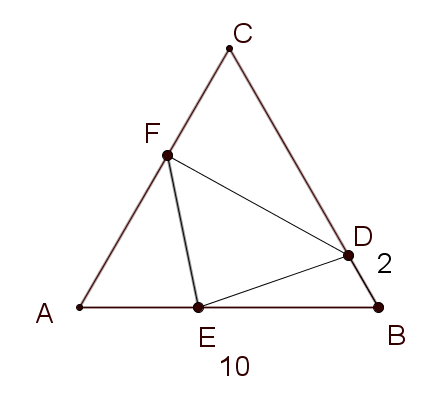# Minimum perimeter

Geometry Level 5As shown, $ABC$ is an equilateral triangle of side length 10. The points $D, E$ and $F$ on $BC, AB$ and $AC$ respectively with $DB=2$. Let $s= DE+EF+FD$. Find the minimum value of $\lfloor 100s\rfloor$.

×

Problem Loading...

Note Loading...

Set Loading...